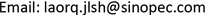1. 罐顶撕裂现象

2. 罐顶撕裂过程2.1. 热油罐基本参数

Main design parameters of the hot oil tan

2.2. 撕裂过程的压力变化

3. 撕裂过程中罐内压力分析

3.1. 油罐体积的计算

3.2. 各时间点罐内压力变化计算3.2.1. 报警

n 1 = P 1 V 3 R T = 101825 × 10458 8.31 × 401 = 319564   mol

3.2.2. 仪表超量程

2:19压力检测仪表已经达到量程最大值，且过该点以后压力一直无法检测到，说明未恢复到量程检测范围内，故在此刻罐内水汽量为：

n 2 = P 2 V 3 R T = 103325 × 10458 8.31 × 401 = 324271   mol

V = ( n 2 − n 1 ) × 18 / ( 60 × 2 ) = 706   g / s

103325 = 101825 + 1500 (变化值)

3.2.3. 呼吸阀超压打开

n 3 = n 2 + 2353   mol / min × 4   min = 333683   mol

P 3 = n 3 R T V 3 = 333683   mol × 8.31 × 401   K 10458 = 106324   Pa

3.2.4. 呼吸人孔打开

2:27作为最后安全屏障的一台呼吸人孔打开，此时罐内水汽总量n4和罐内压力P4的计算应该剔除此时两台呼吸法排出的水汽量Qm

Q = 1.2 ( Q y + V M a x Δ t 237 ε + q r )

Q = 1.2 × 0.0556 = 0.06672   m 3 / s

Q m = 2 ρ Q = 2 × 1415 × 0.06672 = 188.82   g / s

n 4 = n 3 + 706 − 188.82 18 × 4 × 60 = 340579   mol

3.2.5. 人工打开检尺口

2:29分操作人员冲上罐顶，打开检尺口泄压，此时罐内水汽总量n5和罐内压力P5均进一步上升。

n 5 = n 4 + 517.18 − 262.25 18 × 2 × 60 = 342279   mol

P 5 = n 5 R T V 3 = 342279 × 8.31 × 401 10458 = 109063   Pa

3.2.6. 罐顶撕裂

2:33时罐顶撕裂，相比人工打开检尺口后时间过后4分钟，此时罐内水汽总量n6和罐内压力P6为：

n 6 = n 5 + 255 − 23.6 18 × 4 × 60 = 345364   mol

P 6 = n 6 R T V 3 = 110046   Pa

Gas pressure in different period of time (gauge pressure

2:17500
2:192000
2:234999
2:277196
2:297738
2:338721

4. 撕裂过程压力分析

4.1. 压力计算

P V R T = 307714 + 16 x

T为当时最高检测温度128℃ = 401 K

R为气体常数8.314 Pa·m3/mol·K

V为计算出的罐内气相空间10,458 m3

4.2. 实验室数据

4.3. 应力分析

1) 罐顶与槽钢连接焊缝处应力属于圆桶与球面之间无过度焊接，为应力集中点，而且所有储罐设计时又要求该处弱焊，当储罐因受内压较大时，最先撕坏就在此处焊缝，所以筒体与槽钢连接焊缝处应力均大于罐封头和罐筒体应力。

2) 从图4也可以看出最大应力(Von Mises应力)发生在进油管侧的油罐封头与槽钢连接焊缝部位，其最大应力为373 MPa，因此处为进油口，温度最高。

3) 罐底与管壁连接焊缝处应力较罐体应力和罐底应力均大，但较罐顶与槽钢连接焊缝处应力小。

4) 当油罐内压P为8162 Pa (表)时，油罐封头与槽钢连接焊缝处最大应力(最大Von Mises应力)已接近(或达到)材料的抗拉强度，油罐封头与槽钢焊缝连接处发生断裂。

5. 预防措施

1) 严格操作参数的控制。油罐在正常的设计操作情况下(包括呼吸阀、呼吸人孔等正常工作)，不会发生强度破坏。因此，建议油罐的操作压力应不超过油罐的设计压力范围。

2) 油罐罐顶与罐壁之间槽钢(包边角钢)连接焊缝部位(即油罐封头与边梁焊缝部位)发生断裂的主要原因是油罐进油过程中含有大量的水蒸气所致，导致压力急剧升高。因此，操作时严禁进料带水或蒸汽，有轻烃类组分也不得进入热油罐。遇到压力异常波动时应紧急切出物料。

3) 罐顶增加一定直径的呼吸孔用于紧急情况下泄压，可在该油罐罐顶与现有DN500呼吸人孔对面再安装一台上直径为500 mm的呼吸孔，便于紧急泄压和泄压情况下的泄压均匀。

4) 加强油罐呼吸阀和阻火器管理，防止堵塞、锈蚀以及卡死等现象。

5) 增加远程控制阀门，可用于紧急切出或者紧急泄压。

6. 小结